# Derivative. Geometrical and mechanical meaning of derivative

Derivative. Argument and function increments. Differentiable function.
Geometrical meaning of derivative. Slope of a tangent. Tangent equation.
Mechanical meaning of derivative. Instantaneous velocity. Acceleration.

Derivative. Consider a function y = f ( x ) at two points: x 0 and x 0 +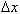: f ( x 0 ) and f ( x 0 +). Heremeans some small change of an argument, called an argument increment ; correspondingly a difference between the two values of a function: f ( x 0 +) – f ( x 0 )  is called a function increment . Derivative of a function y = f ( x ) at a point x 0 is the limit: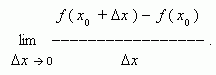If  this limit exists, then a function f ( x ) is a differentiable function at a point x 0 . Derivative of a function f ( x ) is marked as: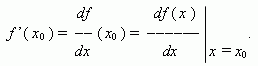Geometrical meaning of derivative. Consider a graph of a function y = f ( x ) :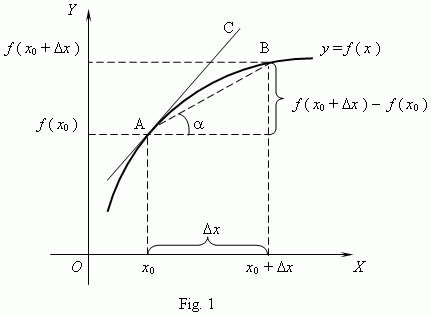From  Fig.1  we see, that for any two points A and B of the function graph: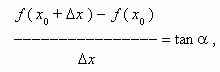where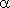- a slope angle of the secant AB.
So, the difference quotient is equal to a secant slope. If to fix the point A and to move the point B towards A, thenwill unboundedly decrease and approach 0, and the secant AB will approach the tangent AC. Hence, a limit of  the difference quotient is equal to a slope of a tangent at point A. Hence it follows: a derivative of a function at a point is a slope of a tangent of this function graph at this point.

Tangent equation. Now we’ll derive an equation of a tangent of a function graph at a point A ( x 0 , f ( x 0 )). In general case an equation of a straight line with a slope f ’( x 0 )  has the shape:

y = f ’( x 0 ) · x + b .

To find b we’ll use the fact, that a tangent line goes through a point A :

f ( x 0 ) = f ’( x 0 ) · x 0 + b ,

hence, b = f ( x 0 ) – f ’( x 0 ) · x 0 ,  and substituting this expression instead of b , we’ll receive the equation of a tangent:

y = f ( x 0 ) + f ’( x 0 ) · ( x – x 0 ) .

Mechanical meaning of derivative. Consider the simplest case: a movement of a material point along a coordinate line, moreover, the motion law is given,  i.e. a coordinate x of this moving point is the known function x ( t ) of  time t . During the time interval from t 0 till t 0 +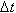the point displacement is equal to: x ( t 0 +) – x ( t 0 ) =,  and its average velocity is: v a =/. As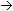0, then an average velocity value approaches the certain value, which is called an instantaneous velocity  v ( t 0 ) of a material point in the moment t 0 . But according to the derivative definition we have: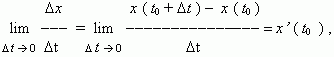hence, v ( t 0 ) = x’ ( t 0 ), i.e. a derivative of a coordinate with respect to time is a velocity. This is a mechanical meaning of a derivative. Analogously to this, an acceleration is a derivative of a velocity with respect to time : a = v’ ( t ).